Courses

# Test: Ideal Gas Equation And Dalton's Law Of Partial Pressure

## 16 Questions MCQ Test Chemistry for JEE | Test: Ideal Gas Equation And Dalton's Law Of Partial Pressure

Description
This mock test of Test: Ideal Gas Equation And Dalton's Law Of Partial Pressure for JEE helps you for every JEE entrance exam. This contains 16 Multiple Choice Questions for JEE Test: Ideal Gas Equation And Dalton's Law Of Partial Pressure (mcq) to study with solutions a complete question bank. The solved questions answers in this Test: Ideal Gas Equation And Dalton's Law Of Partial Pressure quiz give you a good mix of easy questions and tough questions. JEE students definitely take this Test: Ideal Gas Equation And Dalton's Law Of Partial Pressure exercise for a better result in the exam. You can find other Test: Ideal Gas Equation And Dalton's Law Of Partial Pressure extra questions, long questions & short questions for JEE on EduRev as well by searching above.
QUESTION: 1

Solution:
QUESTION: 2

### A gas at a pressure of 5.0 bar is heated from 0°C to 546°C and is simultaneously compressed to one-third of its original volume. Hence, final pressure is

Solution:

P1V1/T1 = P2V2/T2
Let the original volume be 3 volume units . The final volume will be 1/3 of 3 which is 1 volume unit
Temperature must be in K - 0 DEG C = 273K and 546 DEG C = 819 K
We want to solve for P1
P1*1/819 = 5*3/273
P1 = 819*5*3/273
P1 = 45

QUESTION: 3

### A 8.3 L cylinder contains 128 . 0 g O2 gas at 27°C. What mass of O2 gas must be released to reduce the pressure to 6.0 bar ?

Solution:

By applying ideal gas eqn. PV = nRT
Further solving it we get an eq.
w = PVM/RT
Putting values, we get 64g.
Hence, the correct answer is Option A.

QUESTION: 4

N2 gas under the given state I is to be changed to state II. Thus, N2 escaped from I isSolution:

Applying pV = nRT in state 1, we have n1 = 0.110
Applying pV = nRT in state 1, we have n2 = 0.0184
Mole fraction(this fraction is present) = 0.0184/0.110 = 0.16727
So, fraction escaped = (1-0.16727)*100 = 83.33%

QUESTION: 5

1 g H2 gas and x g O2 gas exert a total pressure of 5 atm. At a given temperature partial pressure of O2 gas is 4 atm. This O2 in the mixture is

Solution:
QUESTION: 6

The average molar mass of the vapour above solid NH4CI is nearly 26.75 g mol-1.

Thus, vapour consists of (mole fraction)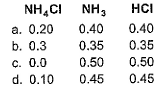Solution:

The correct answer is Option C.

The average molar mass of the vapour above solid NH4Cl = 26.5 mol-1
Evaporation product of NH4Cl --->
NH4Cl (s) ----> NH3(g) + HCl(g)
Let Mole fraction of NH3 = XL
Mole fraction of HCl = X2
∴ Average molar mass of NH3 = 14 + 31 = 17
=> 26.5 = m1x1 + m2x2
=> 26.5 = 17x1 + 36.5x2    ---(i)
And we know;
x1 + x2 = 1
From (i) and (ii)
x1 = 0.5 and x2 = 0.5

QUESTION: 7

Pressure of 1 g of an ideal gas A at 300 K is found to be 2 bar. When 2 g of another ideal gas B is introduced in the same flask at the same temperature, pressure becomes 3 bar. Thus,

Solution:

Given pressure(P) of gas A= 2bar Total pressure = 3bar then pressure(P) of gas B = 3-2 =1bar
no. of mole (n) = given mass(m) / molar mass(M)
Gas A
PV = nRT
2×V =( mₐ/Mₐ) RT ------------- (1)
Gas B
PV = nRT
1V = (mb/Mb)RT ---------------- (2)
On dividing eqn 1 by eqn 2
2V/1V = (1/Mₐ) ÷(2/Mb)
2/1 = (Mb/Mₐ) × 1/2
(MB/Mₐ) = (2/1)×(2/1)
MB/Mₐ = 4/1
MB = 4Mₐ

QUESTION: 8

Total pressure of a mixture of H2 and O2 is 1.00 bar. The mixture is allowed to react to form water, which is completely removed to leave only pure l-l2 at a pressure of 0.35 bar. Assuming ideal gas behaviour and that all pressure measurements were made under the same temperature and volume conditions, the composition of the original mixture is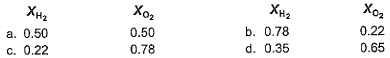Solution:

Correct answer is option B
Avagadro′s Law says:
Equal volume of two gases at same pressure and temperature conditions have equal number of molecules.
∴1 O2 molecule consumes 2 H2 molecules.
∴Since after the reaction only H2 is left,  we know all of O2 is consumed.
∴This implies what all consumed 1/3 of it was O2.
Hence, O2 in the mix was at a partial pressure of (1 - 0.35)* 1/3 = 0.22
And rest was H2 = (1 - 0.22) = 0.78
Hence oxygen- hydrogen composition in the original mix was:0.22 bar O2 and 0.78 bar H2.

QUESTION: 9

The composition of air inhaled by a human is 21 % by volume O2 and 0.03% CO2 and that of exhaled air is 16% O2 and 4.03% CO2. Assuming a typical volume of 6200 L day -1, moles of O2 used and CO2 generated by the body at 37°C and 1.00 bar are (assume ideal behaviour)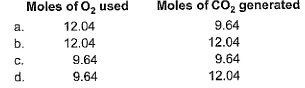Solution:
QUESTION: 10

The following arrangement of flasks is set up. Assuming no temperature change, determine the final pressure inside the system after all stopcocks are opened. The connecting tube has zero volume.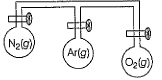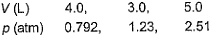Solution:

Applying p1V1 + p2V2 + p3V3 = pnet × Vnet
(0.792)×4 + (1.23)×3 + (2.51)×5 = pnet × 12
On solving, we get p = 1.617 = 1.62 atm

QUESTION: 11

1.00 mole sample of O2 and 3.00 moles sample of H2 are mixed isothermally in a 125.3 L container at 125°C. Gaseous mixture undergoes reaction to form water vapours. Assume ideal gas behaviour total pressure before and after H2O (g) is formed is are respectivel

Solution:

Correct option is not provided.
2H2     +    O2 ------>   2H2O
3         1                0
(-)   2         1                2 mole H2O formed
___________________________
1               0              Total mole = 1+2 = 3

PV = nRT / 100
n = PV / RT
P = nRT/ V
= [4 * 0.083 * (125 + 273)] / 125.3
Pressure = 1.0545 bar after water formed is 2 mole.
P = [3 * 0.083 * 398] /125.3
= 0.7909 bar
= 0.791 bar (appx)
Hence, the answer is 1.0545 bar, 0.7909 bar.

QUESTION: 12

When NO2 is cooled to room temperature, some of it reacts to form a dimer N2O4 through the reaction,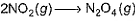In a reaction, 15.2 g of NO2 is placed in a 10.0 L flask at high temperature and the flask is cooled to 25°C. The total pressure is measured to be 0.500 atm. Thus, partial pressure of NO2 is found to be

Solution:

nNO2 + 2nN2O4= 0.330 mol
Subtracting nNO2+ nN2O4 = 0.204 mol
From nNO2+ 2nN2O4 = 0.330 mol
nNO2 = 0.126 mol
nN2O4 = 0.078 mol
From these results, NO2 has a mole fraction of 0.62and a partial pressure of 0.62(0.50 atm) = 0.31 atm;N2O4has a mole fraction of 0.38 and a partial pressure of 0.38(0.50 atm) = 0.19 atm

*Answer can only contain numeric values
QUESTION: 13

Direction (Q. Nos. 13 and 16) This section contains 4 questions. when worked out will result in an integer from 0 to 9 (both inclusive)

Q.
A toy balloon originally held 1.00 g helium gas and had a radius of 10.0 cm.
During the night 0.25 g of the gas effused from the balloon. Assuming the ideal gas behaviour under the constant pressure and temperature conditions, what was the radius of the balloon the next morning? (Express result in cm.)

Solution:

We know that
pV = nRT
A/Q , p and T are constant;
So  n1/n2 = V1/V2
(¼)/(0.25/4) = (10)3/r3
r3 = 750
r  ∼ 9

*Answer can only contain numeric values
QUESTION: 14

What will be the temperature difference needed in a hot air balloon to lift 1.00 kg of mass ? Assume that the volume of the balloon is 100 m3, the temperature of the ambient air is 298 K, the pressure is 1.00 bar, and the air is an ideal gas with average molar mass of 29 g mol-1.

Solution:

The correct answer is 2
The solution is as follows:
Δm = mcold − mhot
=[ncold−nhot]M
= PVMR[1T1− 1T2]
Solving we get:
T2−T1 = ΔT = 2

*Answer can only contain numeric values
QUESTION: 15

Iron forms a series of compounds of the type Fex (CO)y. In air, they are oxidised to Fe2O3 and CO2 gas. After heating a 0.142 g sample in air, you isolate the CO2 in a 1.50 L flask at 25°C. The pressure of the gas is 44.9 mm Fig. What will be the value of y/x  in the simplest empirical formula of Fex(CO)y? [Atomic mass of Fe = 56]

Solution:

The empirical formula is = Fe(CO)2
Carbon dioxide obtained:
Pressure = 44.9 mm Hg
Also, P (mm Hg) = P (atm) / 760
Pressure = 44.9 / 760 = 0.0591 atm
Temperature = 25 °C
The conversion of T( °C) to T(K) is shown below:
T(K) = T( °C) + 273.15
So,
T₁ = (25 + 273.15) K = 298.15 K
V = 1.50 L
Using ideal gas equation as:
PV=nRT
where,
P is the pressure
V is the volume
n is the number of moles
T is the temperature
R is Gas constant having value
= 0.0821 L.atm/K.mol
Applying the equation as:
0.0591 atm × 1.50 L
= n × 0.0821 L.atm/K.mol × 298.15 K
⇒n = 0.0036 moles
1 mole of carbon atoms are present in 1 mole of carbon dioxide. So,
Moles of C = 0.0036 moles
Molar mass of C atom
= 12.0107 g/mol
Mass of C in molecule
= 0.0036 x 12.0107 = 0.0432 g
Given that the compound only contains iron and carbon. So,
Mass of Fe in the sample
= Total mass - Mass of C
Mass of the sample = 0.142 g
Mass of Fe in sample
= 0.142 g - 0.0432 g = 0.0988 g
Molar mass of Fe = 55.845 g/mol
Moles of Fe
= 0.0988  / 55.845  = 0.0018 moles
Taking the simplest ratio for Fe and C as:
0.0018 : 0.0036
= 1 : 2

*Answer can only contain numeric values
QUESTION: 16

To an evacuated vessel with movable piston under external pressure of 1 atm, 0.1 mole of Fie and 0.1 mole of an unknown compound (vapour pressure 0.68 atm at 0°C) are introduced. Considering the ideal gas behaviour, the total volume (in litre) of the gases 0°C is close to...

[IITJEE 2011]

Solution:

Let nx mole of unknown compound be present in vapour form so its partial pressure is
0.68 = (nx/nx+0.1)×1 atm
nx+0.1/nx = 1/0.68 = 1.47
0.1/nx = 0.47
nx = 0.212
Applying ideal gas equation,
1×V = (0.212/0.1)×0.0821×273
V = 7